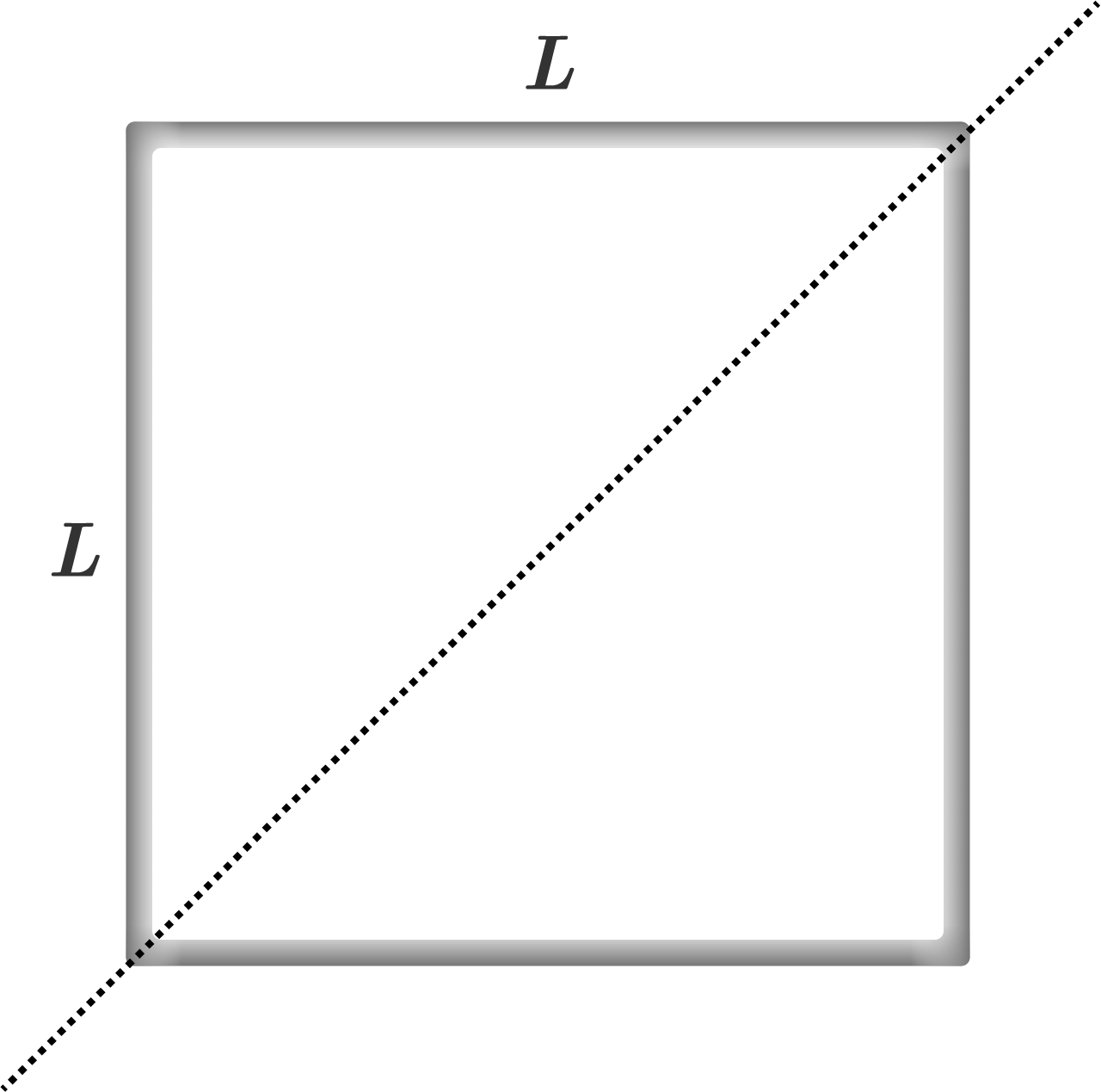# Square Wire MomentConsider a square wire with side length $L$, total mass $M$, and uniform mass density. Determine the wire's moment of inertia about an axis lying in the plane of the square and coinciding with the square's diagonal.

If its value can be represented as $\large{\frac{M L^2}{\alpha}}$, determine the value of $\alpha$.

×